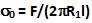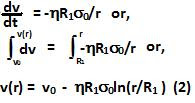## Thursday, July 2, 2009

### Irodov Problem 1.332Let us assume that the shearing force pushing the inner cylinder is F acting in the direction of the axis. Suppose the length of the cylinder is l. The shearing stress at this inner cylinder is given by. At a distance of r from the axis the shear stress will beFrom the basic law of viscosity we can determine the gradient of fluid velocity at a distance r as ,We know that the velocity of the fluid at surface of the outer cylinder is 0, in other words v(R2)=0, hence from (2) we have,